What is the molar mass of calcium chloride?Duc

Concept:

The mass of one mole of a substance in grams is called molar mass. It is equal to the sum of the mass of moles of each element present in the substance. The S.I unit of molar mass is.

Solution:

The steps to calculate the molar mass of a substance are as follows:

1. Write the chemical formula of the substance.

2. Determine the moles of atoms of each element present in one mole of the substance.

3. Take the sum of the mass of moles of each element present in the substance.

The chemical formula of calcium chloride is,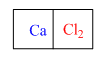The elements present in calcium chloride are:

1. Calcium (Ca)

2. Chlorine (Cl)

In one mole of calcium chloride, one mole of calcium atoms and two moles of chlorine atoms are present.

The mass of one mole of calcium atoms is,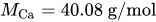The mass of one mole of chlorine atoms is,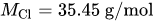The formula to calculate the molar mass of calcium chloride is,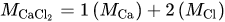…… (1)

Substitute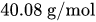for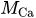and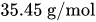for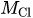in equation (1).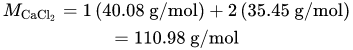Therefore, the molar mass of calcium chloride is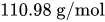.

Similar problems:

Question:

1. What is the molar mass of water?

Solution:

The chemical formula of water is,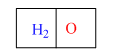The elements present in water are:

1. Hydrogen (H)

2. Oxygen (O)

In one mole of water, two moles of hydrogen atoms and one mole of oxygen atoms are present.

The mass of one mole of hydrogen atoms is,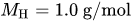The mass of one mole of oxygen atoms is,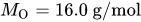The formula to calculate the molar mass of water is,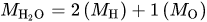…… (2)

Substitute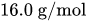for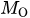and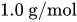for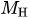in equation (2).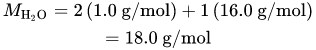Therefore, the molar mass of water is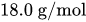.

Question:

2. What is the molar mass of sodium chloride?

Solution:

The chemical formula of sodium chloride is,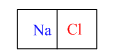In one mole of sodium chloride, one mole of sodium atoms and one mole of chlorine atoms are present.

The mass of one mole of sodium atoms is,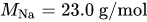The mass of one mole of chlorine atoms is,The formula to calculate the molar mass of sodium chloride is,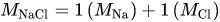…… (3)

Substitute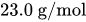for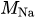andforin equation (3).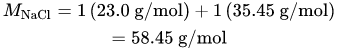Therefore, the molar mass of sodium chloride is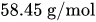.

Need homework help now?

tutors online

Related Questions
2. (11 marks) a) At 400 °C, ammonium iodide decomposes in the following endothermic reaction: NH41(s) + NH3(g) + HI(g) Kp = 0.215 at 400 °C. Write the equilibrium expression for this reaction. 15.0 g of NH4I was placed in an empty 3.0 L flask and heated to 400 °C. What is the partial pressure of NH3 in the flask once the reaction has reached equilibrium? How would the following changes to the equilibrium mixture affect the number of moles of NH3 in the flask and the value of K, once equilibrium is re-established? (Use increase, decrease or stay the same.) Change Effect on moles of NH3 Ke value Increase temperature Increase volume of flask Remove half of HI gas
Notes
Me

OR

Don't have an account?

Join OneClass

Access over 10 million pages of study
documents for 1.3 million courses.

Join to view

OR

By registering, I agree to the Terms and Privacy Policies
Just a few more details

So we can recommend you notes for your school.